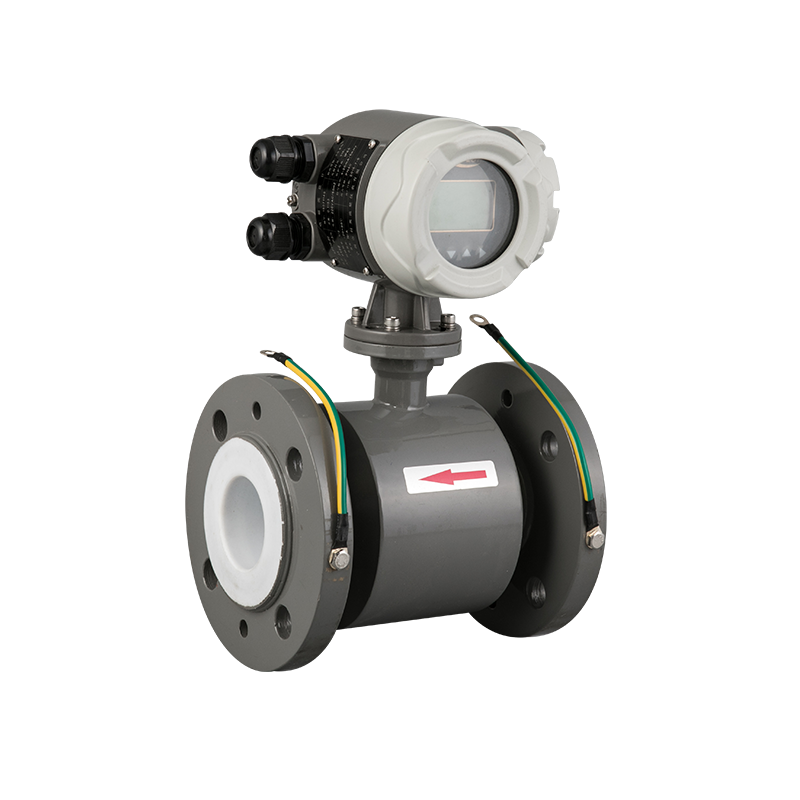###### +86-574-62503881
Inicio / News / What is the calculation method of the diameter of the electromagnetic flowmeter?

# What is the calculation method of the diameter of the electromagnetic flowmeter?Posted by : admin / Posted on : Mar 29， 2021

Electromagnetic flowmeters are mainly used to measure the volume flow of conductive liquids in closed pipelines. Electromagnetic flowmeters stipulate that the minimum flow rate of the fluid is not less than 0.5m/s, normally 2~4m/s, and the highest is not higher than 8m/s. Therefore, when selecting the diameter of the electromagnetic flowmeter, we must fully consider the selection of the appropriate pipe size while ensuring the measurement accuracy of the electromagnetic flowmeter. Then how to determine the diameter of the electromagnetic flowmeter? Let me briefly introduce how to determine the diameter of the electromagnetic flowmeter?
I suppose that there is a 500m³ pool of water that needs to be drained by a pump within 4 hours. How do I determine the diameter of the pipeline to be used? The flow rate range of the flowmeter can be determined by the above-required parameters: 500m³ divided by 4 hours is 125m³/h. The approximate range of the pipe diameter can be calculated by the flow rate, that is: πr²×velocity (0.5~8m/s)=125m³/h. Through calculation, it is known that 125m³/h of water needs to be pumped. The diameter range is 0.075m~0.2975m, which is DN80 Between ~DN300, taking into account the accuracy requirements of the electromagnetic flowmeter, the best flow rate is 2~4m/s, and the caliber is calculated to be 0.105m~0.149m, that is, DN100~DN150. Considering investment and other factors, I can determine that DN100 is more suitable.